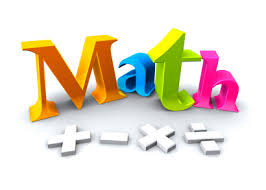• Save

# Maths questions series #3

3 years ago 63.6K Views## Very important Maths questions for SSC CGL-13 Series#3

41. If a number multiplied by 25% of itself gives a number which is 200% more than the number, then the number is –

a. 12
b. 16
c. 35
d. 24

42. The value of an article depreciates every year at the rate of 10% of its value. If the present value of the article is Rs.729, then its worth 3 years ago was-

a. Rs.1250
b. Rs.1000
c. Rs.1125
d. Rs.1200

43. The price of onions has been increased by 50%. In order to keep the expenditure on onions the same the percentage of reduction in consumption has to be-

a. 50%
b. 33 1/3%
c. 33%
d. 30%

44. A took two lo

45. If a regular polygon has each of its angles equal to 3/5 times of two right angles, then the number of side is-

a. 3
b. 5
c. 6
d. 8

46. A square is of area 200 sq. m. A new square is formed in such a way that the length of its diagonal is ?2 times of the diagonal of the given square. The the area of the new square formed is-

a. 200?2 sq.m
b. 400?2 sq.m
c. 400 sq.m
d. 800 sq.m

47. The heights of a cone, cylinder and hemisphere are equal. If their radii are in the ratio 2 : 3 : 1, then the ratio of their volumes is-

a. 2 : 9 : 2
b. 4 : 9 : 1
c. 4 : 27 : 2
d. 2 : 3 : 1

48. A motor-boat can travel at 10 km/hr in still water. It travelled 91 km downstream in a river and then returned to the same place, taking altogether 2 hours. Find the rate of flow of river-

a. 3 km/hr
b. 4 km/hr
c. 2 km/hr
d. 5 km/hr

49. A man driving at 3/4th of his original speed reaches his destination 20 minutes later than the usual time. Then the usual time is-

a. 45 minutes
b. 60 minutes
c. 75 minutes
d. 120 minutes

50. A motor-boat, travelling at the same speed, can cover 25 km upstream and 39 km downstream in 8 hours. At the same speed, it can travel 35 km upstream and 52 km downstream in 11 hours. The speed of the stream is –

a. 2 km/hr
b. 3 km/hr
c. 4 km/hr
d. 5 km/hr